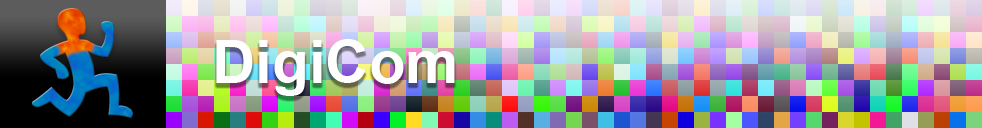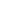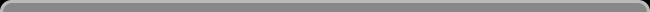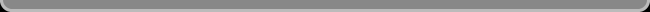Navigation: LaesieWorksImage Compression HomepageThis pageBITS & COMBINATIONS

 A bit All digital computer data (texts, photos, music, applications, ... ) is stored in computer memory (RAM, Hard Disk, CD, DVD, BlueRay disc, USB stick, ...) as "bits". But what are bits? Zeros and Ones? Not really. If you look through a microscope at the surface of a Hard Disk, you will not find very small numbers written on it. A bit is a "yes" or a "no": yes or no electrons (RAM memory), yes or no light reflecting (Optical disc), yes or no positive magnetic force (Hard disk). But when talking about bits, a "no" bit we call a "0", and a "yes" bit we call a "1". A bit confusing A group of 8 bits is called a byte. With a "Kilo Byte" people sometimes refere to 1024 bytes and sometimes to 1000 bytes. KB = Kilo Byte, and Kb = Kilo bit (eight times less than a KB), I personally would like to drop all that because it's confusing. I would rather talk about bits only, and use 1000 for a Kilo. How not to read bits It's not like 0 is nothing and 1 is one, not like this: 0 = 0 1 = 1 11 = 2 111 = 3 1111 = 4 That would be very inefficient. Imagine having to write down the number 16777216 The power of combining bits This is the most efficient digital way of storing values: 0 = 0 1 = 1 00 = 2 01 = 3 10 = 4 11 = 5 000 = 6 001 = 7 010 = 8 and so on. Problem with this variable bits length is that it's also necessary to note how long each code is, which makes it less efficient in some situations. Less complex is to use fixed bits length. For example for a gif image with only 8 colors: 000 = color 1 001 = color 2 010 = color 3 011 = color 4 100 = color 5 101 = color 6 110 = color 7 111 = color 8 How to read bits (fixed length) Here an example of a fixed length of 8 bits. The first bit is at the right. When the first bit is a 0, it's value is 0. When it's a 1, it's value is 1. When the second bit is a 0, it's value is 0. When it's a 1, it's value is 2. When the third bit is a 0, it's value is 0. When it's a 1, it's value is 4. And so on: bit #4 can be 0 or 8 bit #5 can be 0 or 16 bit #6 can be 0 or 32 bit #7 can be 0 or 64 bit #8 can be 0 or 128 And to get the total value of these eight bits, simply ad them up: 00000000 = 0 + 0 + 0 + 0 + 0 + 0 + 0 + 0 = 0 11111111 = 128 + 64 + 32 + 16 + 8 + 4 + 2 + 1 = 255 01001011 = 0 + 64 + 0 + 0 + 8 + 0 + 2 + 1 = 75 10110100 = 128 + 0 + 32 + 16 + 0 + 4 + 0 + 0 = 180COMBINATIONS

 One bit can be one out of two: 0 1 Two bits can together be one out of four combinations: 00 01 10 11 Three bits can together be one out of eight combinations: 000 001 010 011 100 101 110 111 The amount of combinations that can be made with a certain amount of bits is: 2 ^ the amount of bits. 2^1 = 2 2^2 = 2x2 = 4 2^3 = 2x2x2 = 8 2^4 = 2x2x2x2 = 16 And so on. Note that every bit extra, simply doubles the amount of combinations: 2^5 = 32 2^6 = 64 2^7 = 128 2^8 = 256 2^9 = 512 2^10 = 1024 2^11 = 2048 2^12 = 4096 And so on. If you want to calculate some yourself, on a calculator search for: Yx or 2 ^ x 256 RGB pixels (a tiny 16x16 pixels image) are together: 16x16x24 = 6144 bits, thus the amount of combinations is: 2^6144 = 337515218214385611849111744886826404774824526 906135437899871151084665003784674132416503098 237599152146135462950863521351172060726207028 052137864298272611904952952097250362148614445 522452710018844423313364638411836590291565871 784390447510515700543762037763420971108976401 193279180123374243133559193596396525678717812 867692707110267091573529243487775155745125792 074301249607450177138114756335603617462402313 721869876332818900039751245174104362327517860 532580309042489897843433735800204290643997239 816292100396861998120170599620408941456496131 418993734579901361101240330822684452997592142 705570206978374447296284704455535973834352324 658581243318792909226969802227356673323935612 163123006229898288296485668393765657478972738 362500087728995623901601898523186831764997784 754495348973021510834485352883671335921984444 102440129030706195384760231210870118216027271 303420623602926941969150307534755710471876215 776777340159710612862662407760001997614594714 449404632930936532020987293576588671350040321 105994753076183364291878222393348636366688603 375160760250238075243288791621421498051541281 795034053907109943737835019482117307228603598 377500903352726158479078471240691411993347222 620555343776990543813708613373374715745484687 439926604757750285722802679941357049938353559 776954426354329461935618210170897366273790711 314839306845326229523988977154913449422161699 445408113466280894218636839379204569968733539 087852205907225185859091066058115321172389416 793465879841323669578913577817597952200345811 734734797262357003215294955397764406926445582 844693488706710559959872030627305252763960269 629167183586298455205564951525135978709279827 857746749297650724851496824627944089261272676 160606239233708393590584348466105015321542211 296240663356982660509758827921559550059544600 994198771387459924535424019936671684941682457 220968010757943089923275886095202444531971821 40416

 Giesbert Nijhuis